﻿ 油缸缓冲装置结构参数对缓冲性能影响分析
 舰船科学技术2020, Vol. 42Issue (2): 132-136PDF

Analysis of cylinder buffering device′s structure parameters to buffering performance effects
KUANG Quan, ZHANG Zhen-hai, ZHU Shi-jian
College of Naval Architecture and Ocean, Naval University of Engineering, Wuhan 430033, China
Abstract: Open and close noise of the hydraulic cylinder driving mechanism is one of the most important factors that affects the vibration and noise performance of ship mechanical equipment. Adding buffering device can reduce the impact vibration effectively during opening-closing process of driving mechanism. This paper focuses on the buffering device of cylindrical variable—throttling area, then derived functional relationship that buffering cavity pressure and piston velocity changes with piston displacement in which case load was considered. After that, the impacts of different structure parameters: unilateral clearance, diameter at large end of buffering cover, cone length and cone angle on buffering performance was analyzed, optimized design scheme of buffering device was also obtained. The results show that: the unilateral clearance is the most influential factors of buffering performance, secondly are diameter at large end of buffering cover and cone length, cone angle has the least influence on buffering performance. After optimized design, the piston velocity near the stroke end point has reduced 98%, this can reduce open and close noise of hydraulic cylinder driving mechanism effectively.
Key words: buffering device     load     structure parameters     buffering performance     vibration and noise
0 引　言

1 缓冲装置数学模型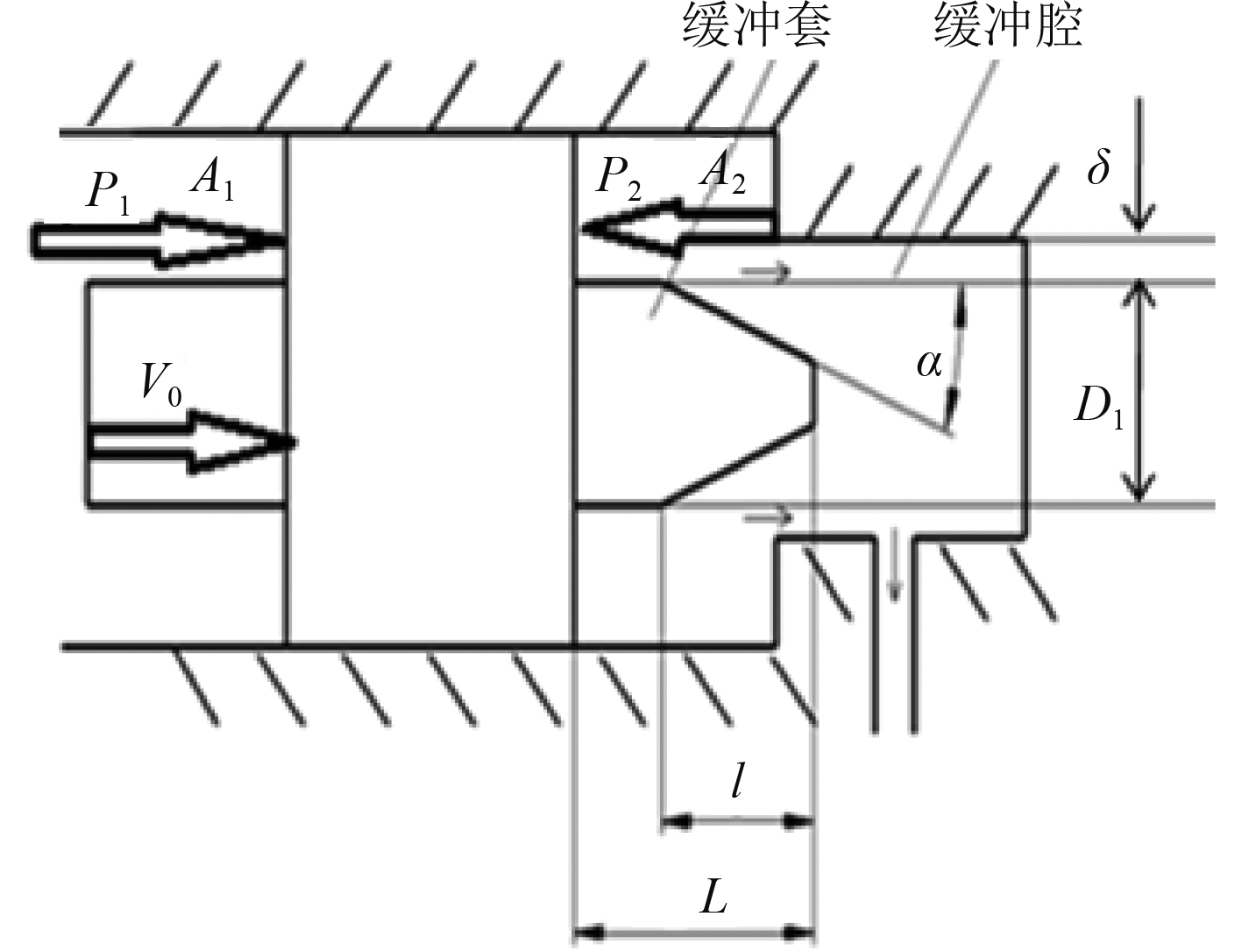图 1 缓冲装置结构简图 Fig. 1 Structure diagram of buffering device

${P_1}$ 为进油压力， ${A_1}$ 为进油压力作用面积， ${A_1} = \dfrac{{\text{π}}}{4}({D^2} - {d^2})$ ${P_2}$ 为缓冲腔压力； ${A_2}$ 为缓冲腔压力作用面积， ${A_2} = \dfrac{{\text{π}}}{4}({D^2} - D_1^2)$ 。式中： $D$ 为液压缸内径；d为活塞杆直径； ${D_1}$ 为缓冲套大端直径。假定油液为不可压缩流体，流动状态为紊流，密封件摩擦阻力相对于惯性力可以忽略不计。以活塞为分析对象，进行受力分析如下：

 ${p_1}{A_1} - {p_2}{A_2} - F = ma {\text{。}}$ (1)

 $Q = {v_x}{A_2} {\text{。}}$ (2)

 $Q = {C_q}A\sqrt {\frac{{2\left| {\Delta p} \right|}}{\rho }} {\text{。}}$ (3)

 $\left| {\Delta p} \right| = \frac{{{Q^2}\rho }}{{2{C_q}^2{A^2}}} = \frac{{{{({v_x}{A_2})}^2}\rho }}{{2{C_q}^2{A^2}}} {\text{。}}$ (4)

 $\begin{array}{l} { A} = \left[ {3\left[ \begin{array}{l} 0.004\;36D_1^2\arccos \left[ {1 - \dfrac{{{L_2}\tan \alpha }}{{{{{D_1}}}/{2}}}} \right] - \\ \dfrac{{{D_1}}}{2}\sin \left[ {\arccos \left[ {1 - \dfrac{{{L_2}\tan \alpha }}{{{{{D_1}}}/{2}}}} \right]} \right] \times \\ \left(\dfrac{{{D_1}}}{2} - {L_2}\tan \alpha \right) \\ \end{array} \right] + {A_3}} \right] \times {10^{ - 6}} {\text{。}} \end{array}$ (5)

1）从缓冲腔压力开始形成到进油压力 ${p_1}$ 达到溢流阀开启压力 ${p_{1\max }}$ 为止，这个过程活塞做匀速运动， ${v_x} = {v_0}$ 。设进油压力 ${p_1}$ 刚好达到溢流阀开启压力 ${p_{1\max }}$ 时，缓冲腔压力为 ${p_{22}}$ ，则 ${p_{22}} = \dfrac{{{p_{1\max }}{A_1} - F}}{{{A_2}}}$ ，与式（4）、式（5）联立可以求出此时对应的 ${L_x}$ ，取为 ${L_{x0}}$ 。根据式（4）得第1阶段缓冲腔压力变化方程如下：

 ${p_2} = \frac{{A_2^2\rho v_0^2}}{{2{C_q}^2{A^2}}} {\text{。}}$ (6)

2）从进油压力 ${p_1}$ 达到溢流阀开启压力 ${p_{1\max }}$ 开始，到缓冲结束，这个过程活塞做变减速运动，加速度 $a$ 不为定值。假设速度函数连续、可导、可局部线性化，计算时把这一段距离分成若干段非常小的 $\Delta {L_x}$ ，则每个小段内，活塞可近似视为作匀减速运动，其对应的加速度为 ${a_x}$ 。设任意小段起始位置的速度为 ${v_{x - 1}}$ $x \geqslant 1$ ），任意小段结束位置的速度为 ${v_x}$ ，则

 ${v_x} = \sqrt {v_{x - 1}^2 - 2{a_x}\Delta {L_x}} {\text{。}}$ (7)

 ${p_2} = \frac{{A_2^2\rho (v_{x - 1}^2 - 2{a_x}\Delta {L_x})}}{{2{C_q}^2{A^2}}} {\text{，}}$ (8)

 ${a_x} = \frac{{2{C_q}^2{A^2}(F - {p_{1\max }}{A_1}) + A_2^3\rho v_{x - 1}^2}}{{2(A_2^3\rho \Delta {L_x} - {C_q}^2{A^2}m)}} {\text{。}}$ (9)

2 参数对缓冲性能影响分析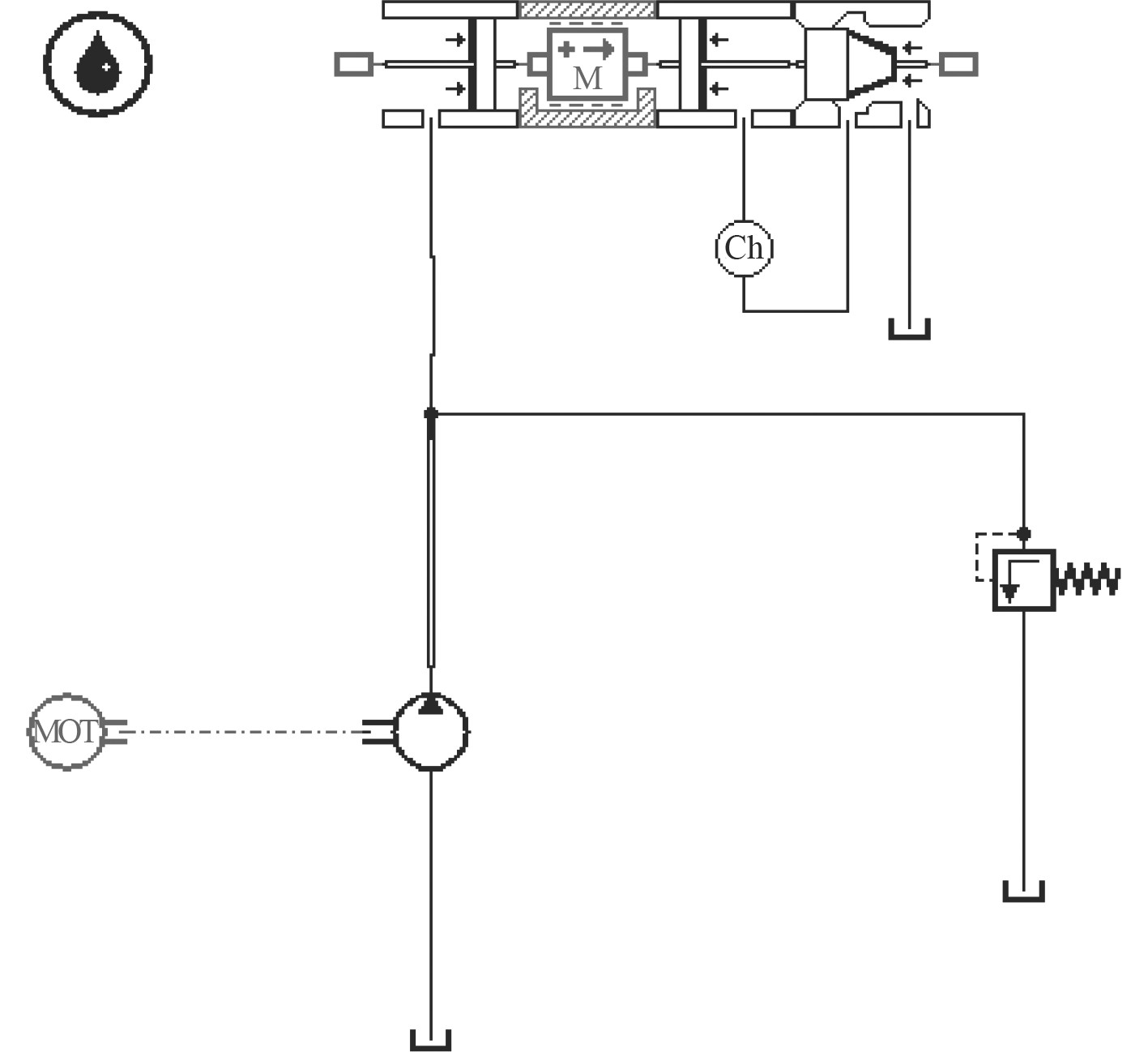图 2 AMESim仿真模型 Fig. 2 Simulation model of AMESim表 1 仿真参数 Tab.1 Simulation parameters

2.1 单边间隙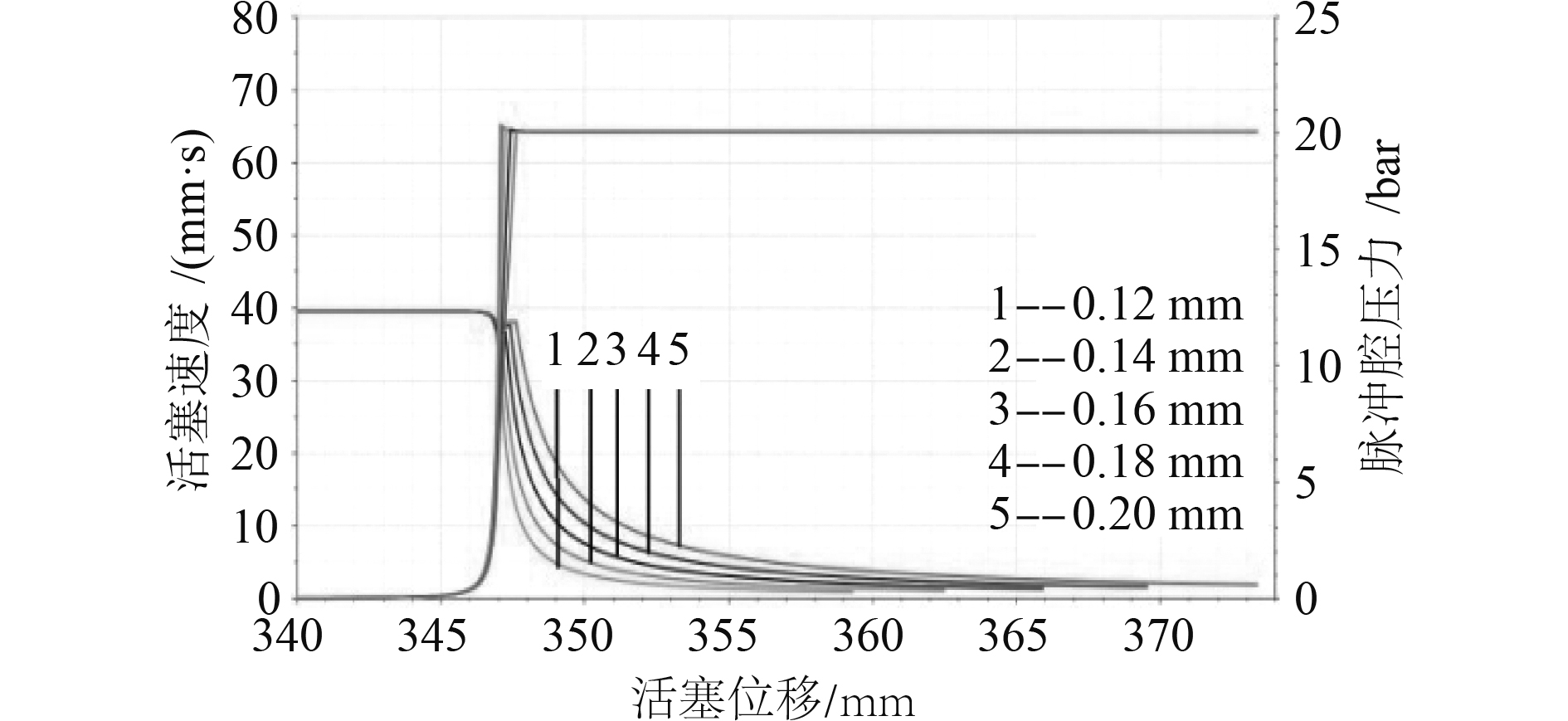图 3 不同单边间隙下活塞速度与缓冲腔压力随活塞位移变化曲线 Fig. 3 Curves of buffering cavity pressure and piston velocity changes with piston displacement under different unilateral clearances

2.2 缓冲套大端直径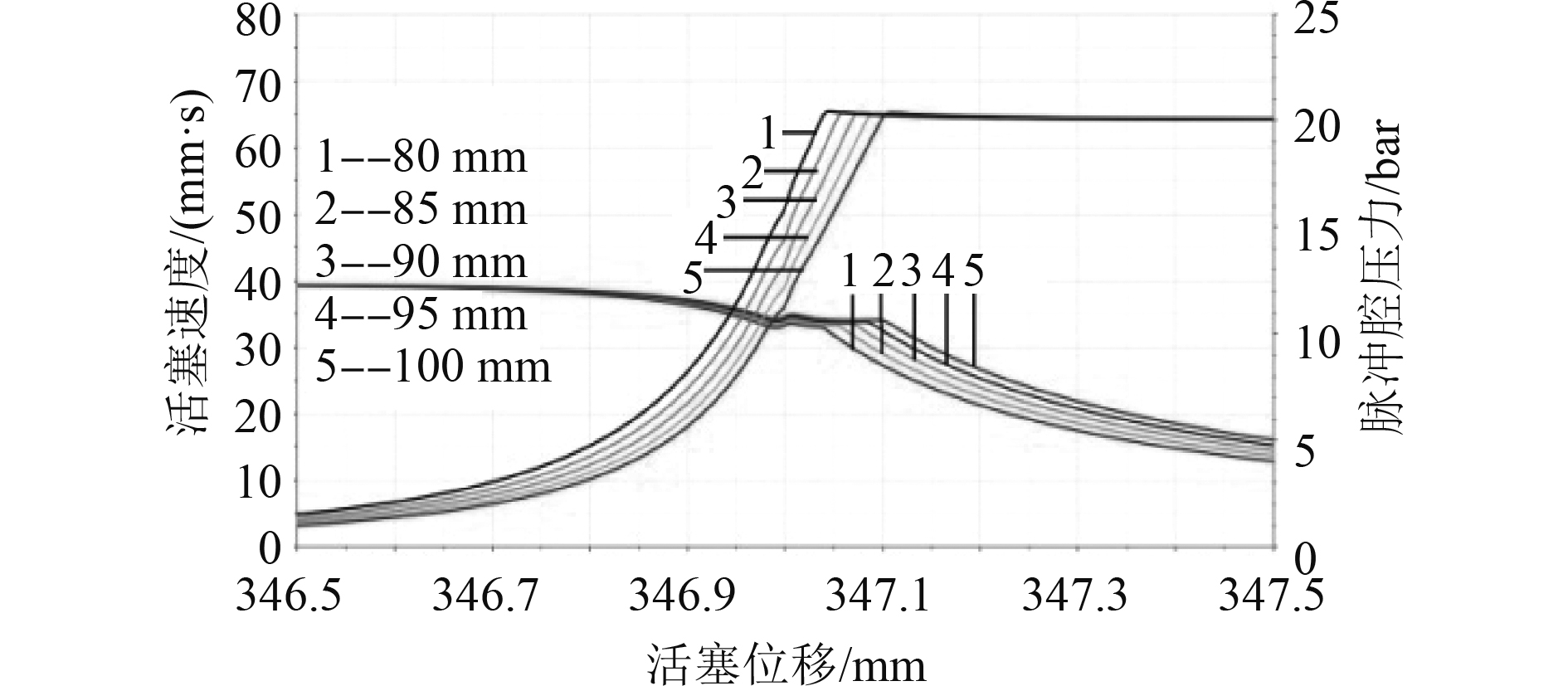图 4 不同缓冲套大端直径下活塞速度与缓冲腔压力随活塞位移变化曲线 Fig. 4 Curves of buffering cavity pressure and piston velocity changes with piston displacement under different diameters at large end of buffering cover
2.3 圆锥段长度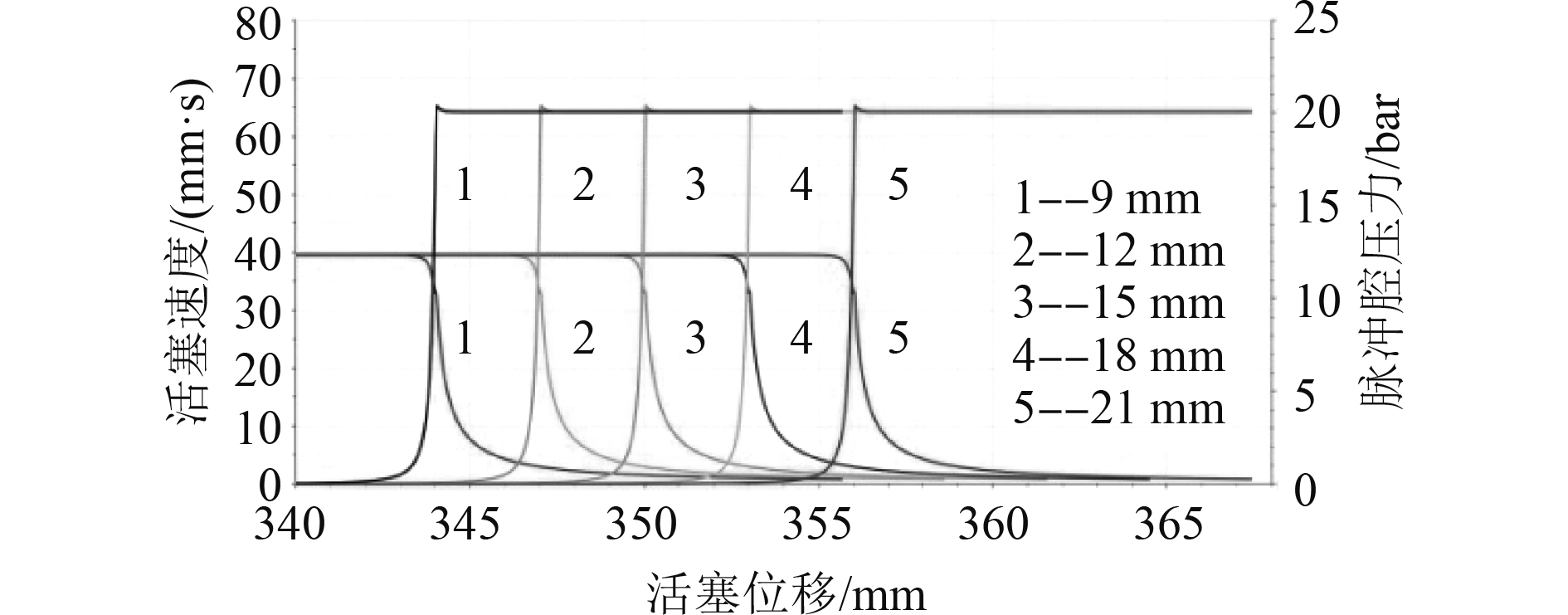图 5 不同圆锥段长度下活塞速度与缓冲腔压力随活塞位移变化曲线 Fig. 5 Curves of buffering cavity pressure and piston velocity changes with piston displacement under different cone lengths
2.4 锥角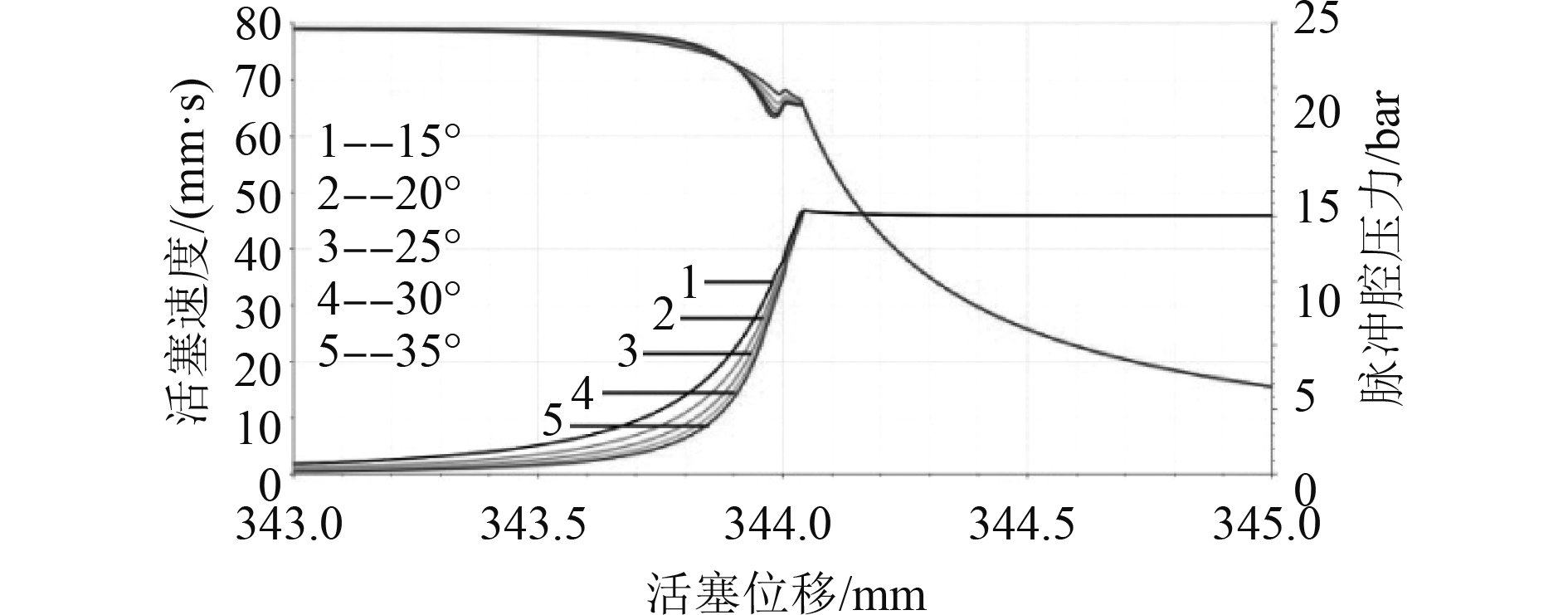图 6 不同锥角下活塞速度与缓冲腔压力随活塞位移变化曲线 Fig. 6 Curves of buffering cavity pressure and piston velocity changes with piston displacement under different cone angles
3 参数优化与缓冲性能分析表 2 缓冲装置结构参数 Tab.2 Structure parameters of buffering device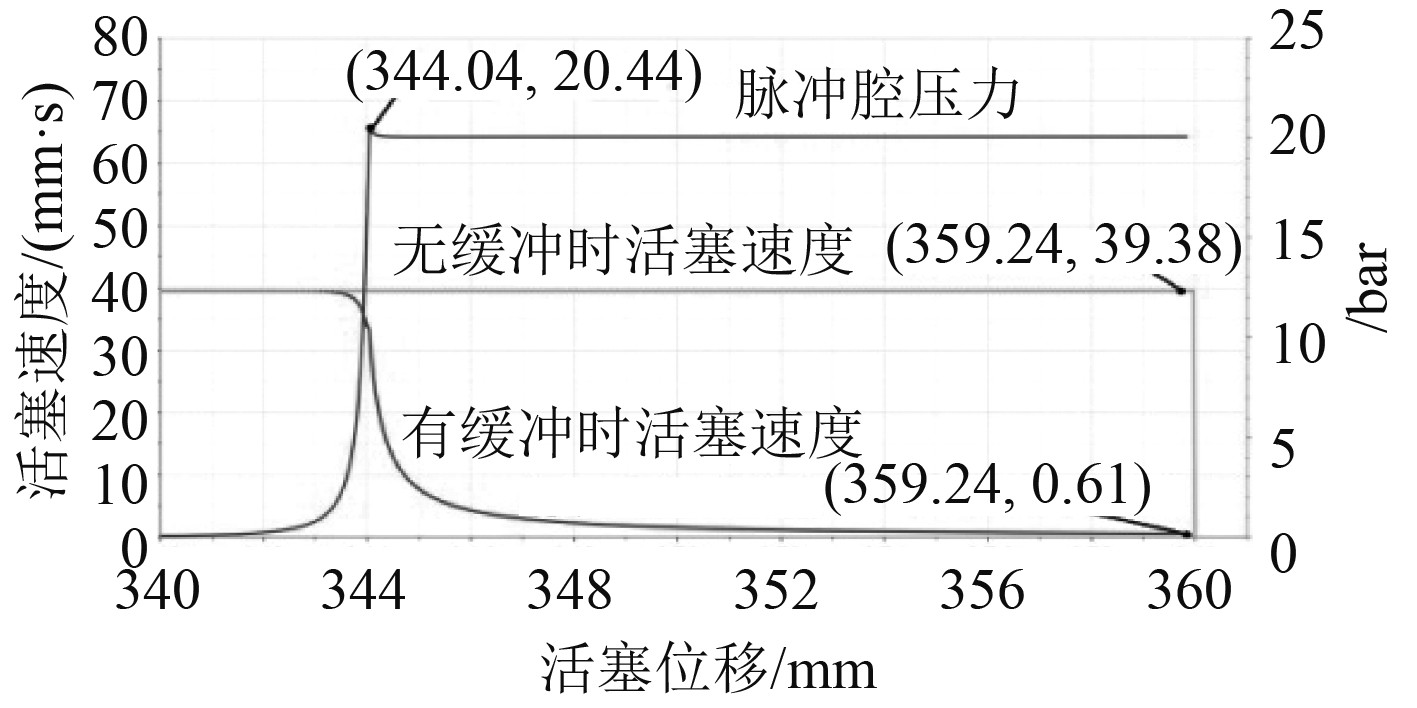图 7 活塞速度与缓冲腔压力随活塞位移 Fig. 7 Curves of buffering cavity pressure and piston velocity changes with piston displacement

4 结　语

1）单边间隙对缓冲腔压力和活塞速度的影响最大，缓冲套大端直径、锥角的影响次之，圆锥段长度对缓冲性能的影响最小。

2）有缓冲与无缓冲相比，活塞在到达行程终点前的速度（盖板与止挡板的撞击速度）下降约为98%，缓冲过程较为平缓，没有出现较大的液压冲击。

  周志才, 吴新跃, 谢最伟. 基于ADAMS/Insight的某传动机构参数优化[J]. 机械工程师, 2011(6): 64-66. DOI:10.3969/j.issn.1002-2333.2011.06.030  赖浩凯, 楼京俊, 孙炯, 等. 基于ADAMS和AMESim软件的舰艇某装置启闭建模及优化[J]. 中国舰船研究, 2014, 9(3): 105-108+122. DOI:10.3969/j.issn.1673-3185.2014.03.016  赵伟, 黄钰曌, 俞浙青, 等. 高速液压缸活塞式缓冲机构的研究[J]. 中国机械工程, 2014, 25(8): 1033-1036. DOI:10.3969/j.issn.1004-132X.2014.08.008  邓经纬, 曹楚君. 基于AMESim油缸缓冲装置优化设计[J]. 机床与液压, 2017, 45(10): 122-125. DOI:10.3969/j.issn.1001-3881.2017.10.032  王传瑶, 王勇勤, 罗远新, 等. 液压缸缓冲动态特性对比研究[J]. 液压与气动, 2017(10): 89-95. DOI:10.11832/j.issn.1000-4858.2017.10.015  吴仁智, 徐君, 张洪伟. 圆柱形变节流面积缓冲结构的缓冲性能分析[J]. 中国工程机械学报, 2013, 11(3): 211-215. DOI:10.3969/j.issn.1672-5581.2013.03.006  梁全, 谢基晨, 聂利卫. 液压系统Amesim计算机仿真进阶教程[M]. 北京: 机械工业出版社, 2016.